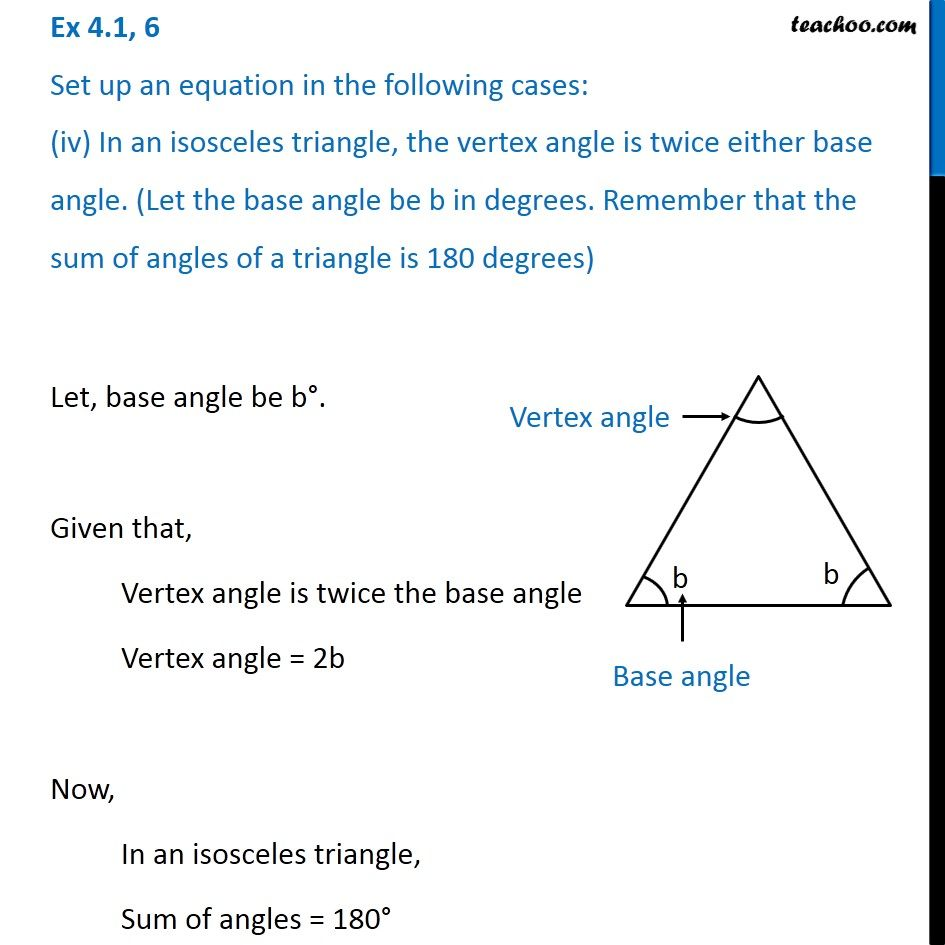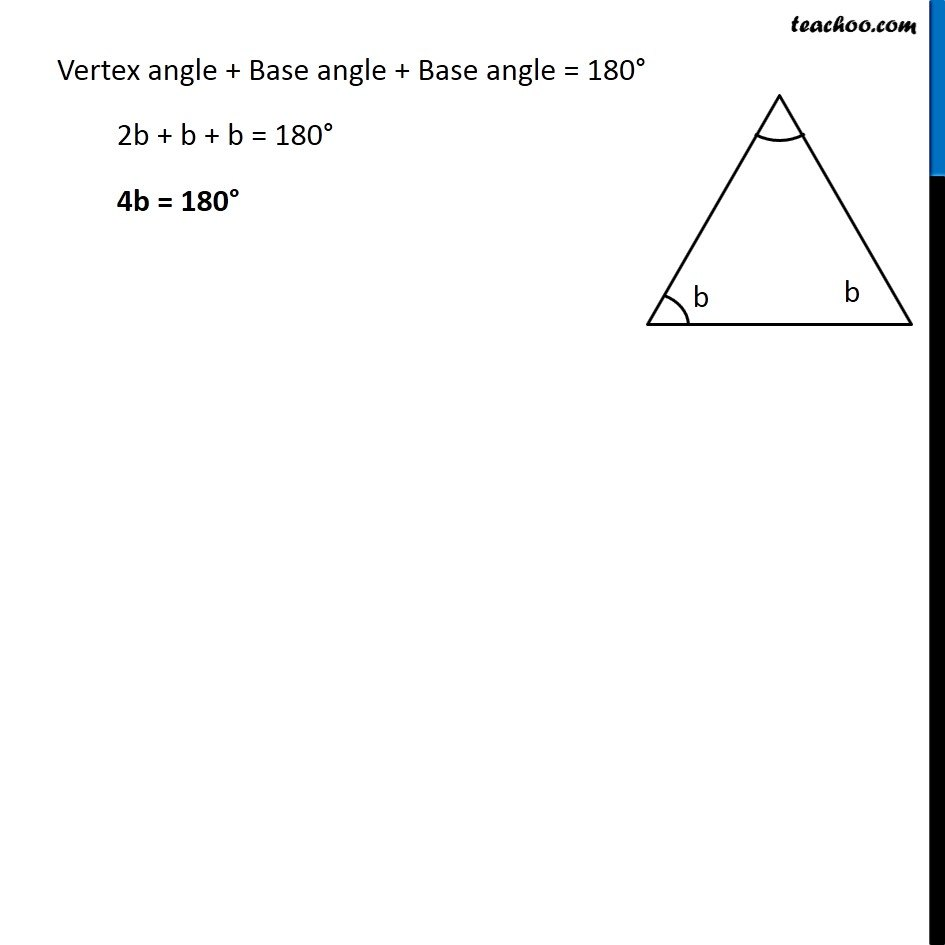Ex 4.1

Chapter 4 Class 7 Simple Equations
Serial order wiseLearn in your speed, with individual attention - Teachoo Maths 1-on-1 Class

### Transcript

Ex 4.1, 6 Set up an equation in the following cases: (iv) In an isosceles triangle, the vertex angle is twice either base angle. (Let the base angle be b in degrees. Remember that the sum of angles of a triangle is 180 degrees) Let, base angle be b . Given that, Vertex angle is twice the base angle Vertex angle = 2b Now, In an isosceles triangle, Sum of angles = 180 Vertex angle + Base angle + Base angle = 180 2b + b + b = 180 4b = 180